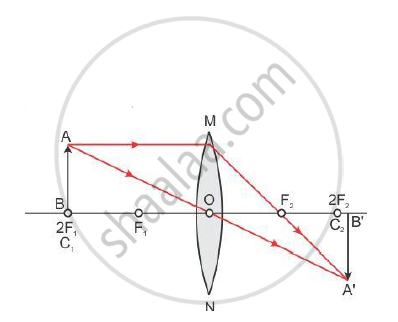# A Student is Using a Convex Lens of Focal Length 10 Cm to Study the Image Formation by a Convex Lens for the Various Positions of the Object. - Science

A student is using a convex lens of focal length 10 cm to study the image formation by a convex lens for the various positions of the object. In one of his observations, he may observe that when the object is placed at a distance of 20 cm from the lens, its image is formed at (select the correct option)

(A) 20 cm on the other side of the lens and is of the same size, real and erect.

(B) 40 cm on the other side of the lens and is magnified, real and inverted.

(C) 20 cm on the other side of the lens and is of the same size, real and inverted.

(D) 20 cm on the other side of the lens and is of the same size, virtual and erect.

#### Solution 1

(C) 20 cm on the other side of the lens and is of the same size, real and inverted.

Focal length f = 10 cm
The object is placed at 2F (2 ⨯ 10 = 20 cm). Hence the image is also formed at 2F.Position of object Position of image Size of image Nature of image At 2F1 At 2F2 Same size Real and inverted

#### Solution 2

Focal length of the lens, f = 10 cm

Object distance, u = −20 cm (negative sign is used due to sign convention)

Using the lens formula:

1/f=1/v-1/u

rArr1/10=1/v-1/(-20)

rArr1/v=1/10-1/20

rArr1/v=(2-1)/20

rArrv=20" cm"

Hence, the image will be formed on the other side of the lens.

Magnification is given as:

m=v/u=h_i/h_o

h_i/h_o=20/(-20)

h_i=-h_o

Therefore, the image formed by the convex lens will be of same size, real and inverted.

Hence, the correct option is C.

Concept: Magnification Due to Spherical Lenses
Is there an error in this question or solution?
2013-2014 (March) All India Set 3

Share